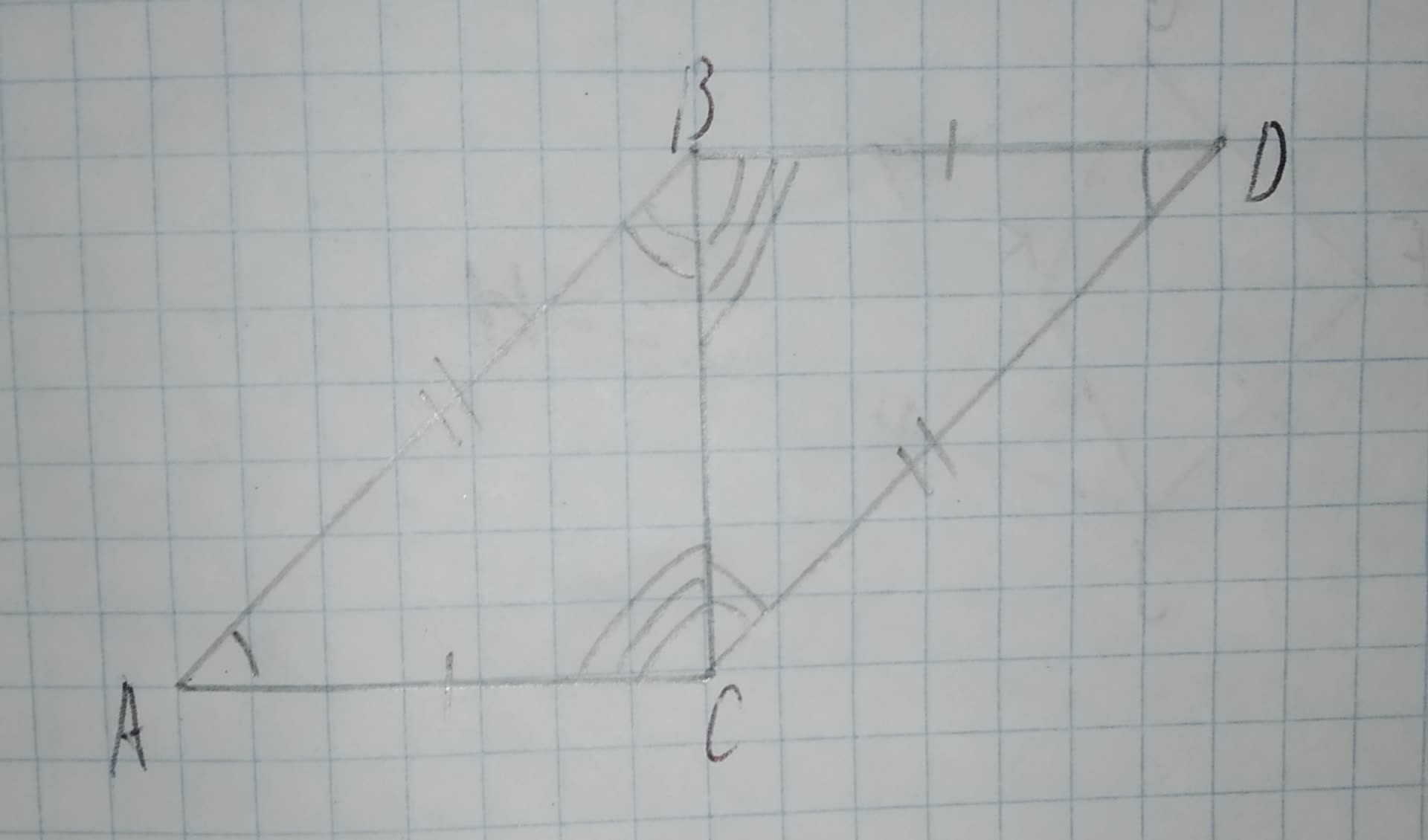# Using the diagram below, complete the following congruence statement: /_ABC ~= ? 12210203771.jpg a)/_DBC b)/_BDC c)/_BCD d)/_DCBChardonnay Felix 2020-12-03 Answered
Using the diagram below, complete the following congruence statement:
$\mathrm{△}ABC\stackrel{\sim }{=}$ ?a)$\mathrm{△}DBC$
b)$\mathrm{△}BDC$
c)$\mathrm{△}BCD$
d)$\mathrm{△}DCB$
You can still ask an expert for help

## Want to know more about Congruence?

• Questions are typically answered in as fast as 30 minutes

Solve your problem for the price of one coffee

• Math expert for every subject
• Pay only if we can solve itSally Cresswell
Step 1
From the figure given that:
Two triangles ABC and DCB
In given figure,
Both triangles have same sides and same angle
So both triangles are congruence.
Step 2
$\mathrm{△}ABC\stackrel{\sim }{=}\mathrm{△}DCB$
Hence option (d) is correct.